# 7.NS.A.1b / 7.NS.A.1c - Addition and Subtraction of Rational Numbers (Part 1 of 2)

##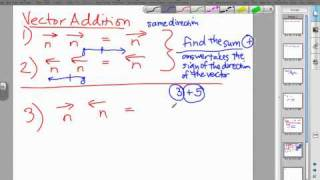By MathwithMrAlmeida

Watch Mr. Almeida fully explain how to add and subtract rational numbers using vectors and the concept of a number line.# Absolute Value and Additive Inverse | 7.NS.A.1 | 7th Grade Math

##By ParksMath# Multiplying and Dividing Decimals | 7.NS.A.2c | 7th Grade Math

##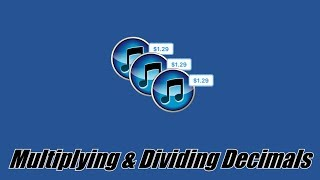By ParksMath | Todd Parks

ParksMath explains how to multiply and divide decimals numbers without the use of a calculator. Understanding how to change a decimal number so that it is an integer, makes multiplying and dividing decimals much easier. This quick tutorial will give you thee tools that you need to find the product or quotient of any decimal number.# Multiplying and Dividing Decimals | 7.NS.A.2c | 7th Grade Math

##By ParksMath

ParksMath explains how to multiply and divide decimals numbers without the use of a calculator. Understanding how to change a decimal number so that it is an integer, makes multiplying and dividing decimals much easier. This quick tutorial will give you thee tools that you need to find the product or quotient of any decimal number.# Multiplying Fractions and Mixed Numbers | 7.NS.A.2a | 7th Grade Math

##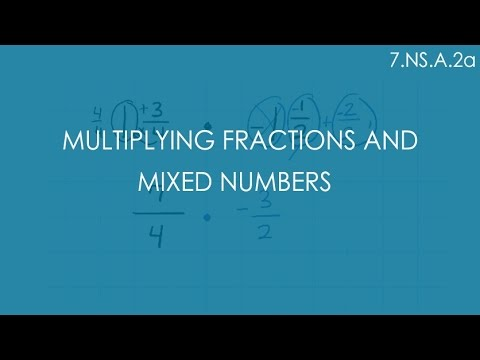By ParksMath

This tutorial shows students how to multipy fractions and mixed numbers. They will learn how to turn mixed numbers into improper fractions and will also see how to apply integer rules when multiplying signed numbers. Simplifying fractions before multiplying is also introduced as a strategy to getting a complete and correct reduced answer.# Additive inverse and distance between two points on a number line

##By Don't Memorise

Absolute value of their difference# Rational Numbers As Quotients of Integers

##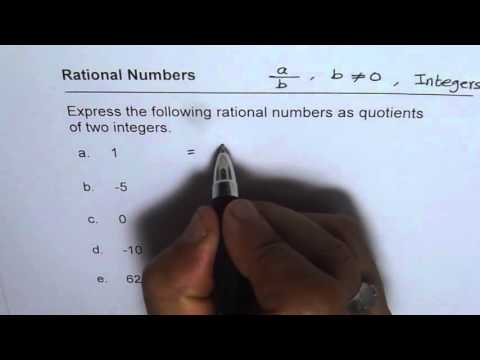By Anil Kumar

every quotient of integers is a rational number.# Division of Rational Numbers

##By Khan Academy

This video walks through the division of 4-5 rational numbers and includes a word problem.# Comparing rational numbers

##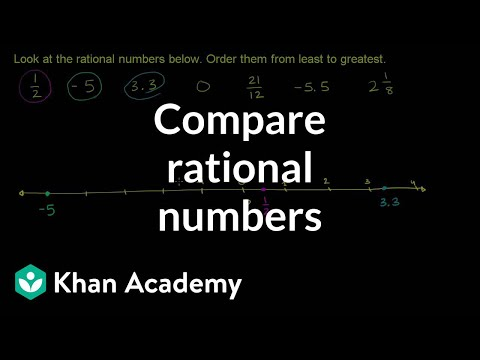By Khan Academy

U09_L1_T3_we4 Comparing Rational Numbers# Dividing mixed numbers

##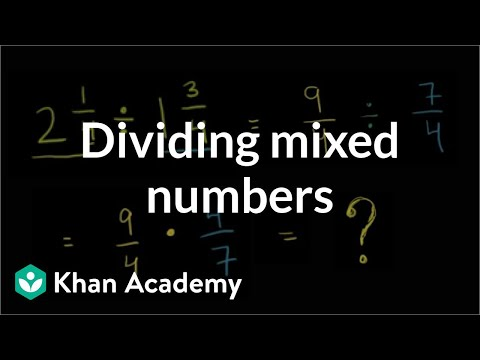By Khan Academy

Dividing Mixed Numbers# Multiplying and dividing negative numbers

##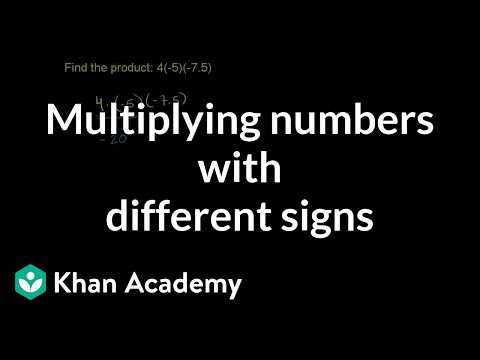By Khan Academy

Find the product of numbers with different signs.# Multiplying and dividing negative numbers

##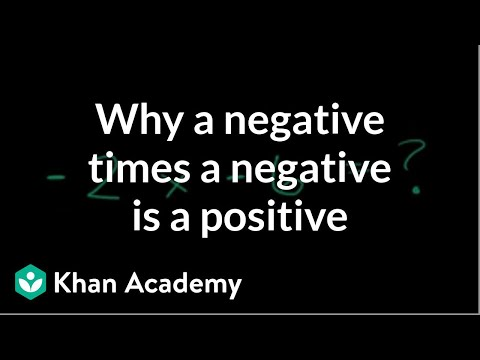By Khan Academy

Use the distributive property to understand the products of negative numbers.# Multiplying and dividing negative numbers

##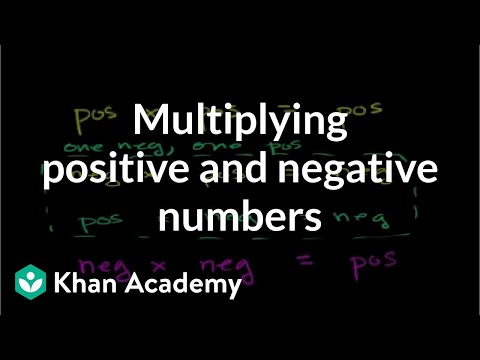By Khan Academy

Learn some rules of thumb for multiplying positive and negative numbers.# Multiplying and dividing negative numbers

##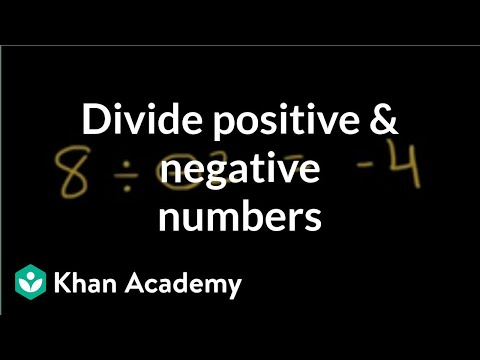By Khan Academy

Discover the basics of dividing with negative numbers.ï¿½ï¿½ï¿½ï¿½ï¿½ï¿½ï¿½ï¿½ï¿½ï¿½ï¿½ï¿½# Multiplying and dividing negative numbers

##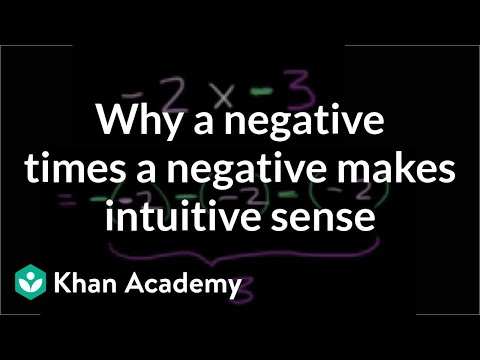By Khan Academy

Use the repeated addition model of multiplication to give an understanding of multiplying negative numbers.# Order of operations with negative numbers

##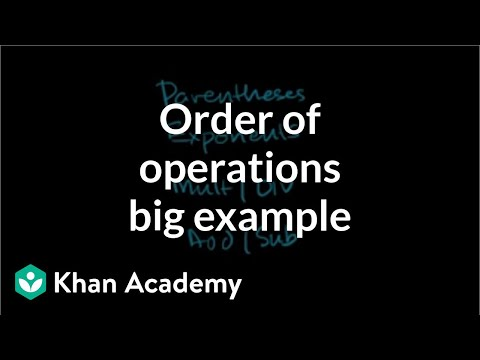By Khan Academy

Simplify this tricky expression using the order of operations. ï¿½ï¿½ï¿½ï¿½ï¿½ï¿½ï¿½ï¿½ï¿½ï¿½ï¿½ï¿½ Expression include negative numbers and exponents.# Order of operations with negative numbers

##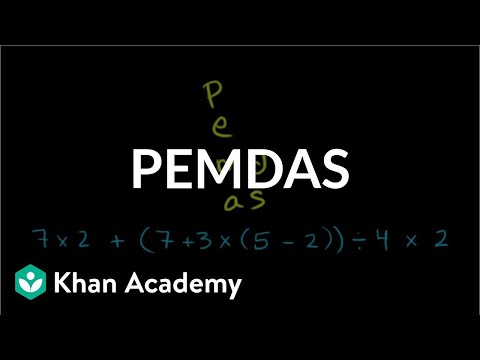By Khan Academy

Work through another challenging order of operations example with only positive numbers.# Order of operations with negative numbers

##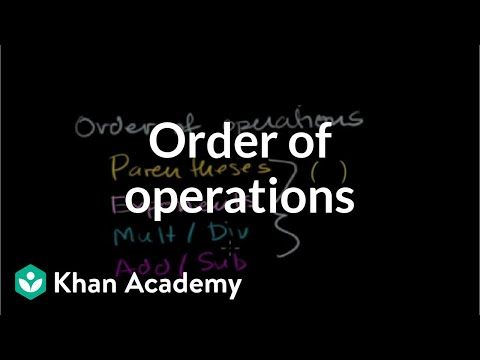By Khan Academy

This example clarifies the purpose of order of operations: to have ONE way to interpret a mathematical statement.# Passive Sentences (Part 1)

##By Smrt English

Mark demonstrates how to make a sentence in the passive voice using 'be + past participle'.# The Active & Passive Voices #1

##By Smrt English

This lesson explains the reasons and ways to use the active and passive voices. Passive sentences shift the focus from the subject to the object. Passive sentences are used when the agent (doer of the action) is not important or unknown or the agent is clear.